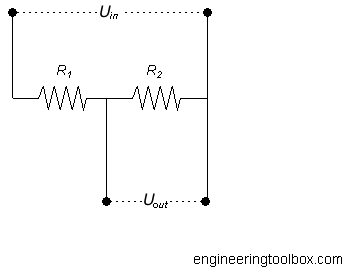Engineering ToolBox - Resources, Tools and Basic Information for Engineering and Design of Technical Applications!

# Potential Divider - Online Calculator

## Output voltage with a potential divider.

A potential divider is the simplest way of producing a source of lower e.m.f. from a source of higher e.m.f.The output voltage from a potential divider can be calculated as

Uout = Uin R2 / (R1 + R2)                 (1)

where

Uout = output voltage (V)

R = resistance (Ohms, Ω)

Uin = input voltage (V)

### Example - Potential Divider - High Power Consumption

The output voltage from a potential divider with two resistors R1 = 10 ohms and R2 = 20 ohms and input voltage 12 V can be calculated as

Uout = (12 V) (20 Ω) / ((10 Ω) + (20 Ω))

= 8 (V)

The current through the potential divider R1 and R2 (ex. output current) can be calculated by using Ohm's law

I = U / R

= (12 V) / ((10 Ω) + (20 Ω))

= 0.4 A

The power consumption of the divider can be calculated

P = U I

= (12 V) (0.4 A)

= 4.8 W

### Example - Potential Divider - Lower Power Consumption

The output voltage from a potential divider with two resistors R1 = 1000 ohms and R2 = 2000 ohms and input voltage 12 V can be calculated as

Uout = (12 V) (2000 Ω) / ((1000 Ω) + (2000 Ω))

= 8 (V)

The current through the potential divider R1 and R2 (ex. output current) can be calculated by using Ohm's law

I = U / R

= (12 V) / ((1000 Ω) + (2000 Ω))

= 0.004 A

The power consumption of the divider can be calculated

P = U I

= (12 V) (0.004 A)

= 0.048 W

The power consumption in a potential divider can be reduced by increased resistance.

### Potential Divider - Calculator

input voltage Uin (volts)

resistor R1 (ohm)

resistor R2 (ohm)

#### Potential Divider Nomogram

The nomogram below can be used to estimate a potential divider.

The default values in the nomogram abocve are for Uin = 12 VR2 = 47 ohm and Uout3.3 V. Since the sum of the resistances (R1 + R2) according the nomogram is approximately 170 ohm - the resistance R1 can be calculated as

R1 ≈ (170 ohm - 47 ohm)

≈ 123 ohm.

## Related Topics

• Electrical - Electrical units, amps and electrical wiring, wire gauge and AWG, electrical formulas and motors.

## Engineering ToolBox - SketchUp Extension - Online 3D modeling!

Add standard and customized parametric components - like flange beams, lumbers, piping, stairs and more - to your Sketchup model with the Engineering ToolBox - SketchUp Extension - enabled for use with the amazing, fun and free SketchUp Make and SketchUp Pro .Add the Engineering ToolBox extension to your SketchUp from the SketchUp Pro Sketchup Extension Warehouse!

Translate

## Privacy

We don't collect information from our users. Only emails and answers are saved in our archive. Cookies are only used in the browser to improve user experience.

Some of our calculators and applications let you save application data to your local computer. These applications will - due to browser restrictions - send data between your browser and our server. We don't save this data.

## Citation

• Engineering ToolBox, (2008). Potential Divider - Online Calculator. [online] Available at: https://www.engineeringtoolbox.com/potential-divider-d_1384.html [Accessed Day Mo. Year].

Modify access date.

. .

#### Scientific Online Calculator6 24